# Difference between revisions of "Contributions:IIRFilter ML"

## Synopsis

A finite impulse response (IIR) filter for temporal filtering that behaves like Matlab's filter() function.

## Versioning

### Authors

Juergen Mellinger

### Source Code Revisions

• Initial development: 6005
• Tested under: 6008
• Known to compile under: 6008
• Broken since: --

## Matlab filter() Function

The Matlab filter() function is a direct form II transposed implementation of the IIR difference equation. If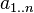$a_{1..n}$ are the denominator coefficients of the filter polynomial, and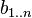$b_{1..n}$ are the numerator coefficients of the filter polynomial, and the shorter of the two is padded with zeros, then the direct form II implementation computes the filter's output at sample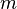$m$ as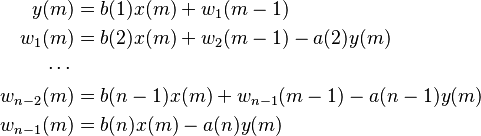\begin{align} y(m) &= b(1) x(m) + w_1(m-1) \\ w_1(m) &= b(2) x(m) + w_2(m-1) - a(2) y(m)\\ \cdots{} \\ w_{n-2}(m) &= b(n-1) x(m) + w_{n-1}(m-1) - a(n-1) y(m)\\ w_{n-1}(m) &= b(n) x(m) - a(n) y(m) \end{align}

where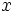$x$ is the input,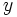$y$ is the output, and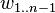$w_{1..n-1}$ are the filter delays.

## Parameters

### NumeratorB

List of numerator coefficients for the filter polynomial. Corresponds to the b parameter of the Matlab filter() function.

### DenominatorA

List of denominator coefficients for the filter polynomial. Corresponds to the a parameter of the Matlab filter() function. The list must contain at least one value, and that value must be nonzero. All coefficients of the filter polynomial will be normalized dividing by the first entry of DenominatorA.

NumeratorB and DenominatorA coefficients will be applied to all channels; it is not possible to specify indiviual coefficients for channels.

### DelaysZi

Matrix of individual initial values of the filter delays. Corresponds to the zi parameter of the Matlab filter() function. Columns correspond to channels, rows correspond to delays.

If empty, filter delays are filled with zeros. At the end of each run, the DelaysZi parameter is updated with the current values of the delays, i.e. the value of the zf parameter in Matlab.

None.